• 2018-10-21 10:54:53

进化算法VS传统数学优化方法

• 进化算法是基于种群的搜索

• 进化算法是随机的搜索算法，不需要梯度，不需要解析的目标函数

进化算法优势

• 适用于没有解析目标函数和无法得到目标函数梯度信息的优化问题

• 基于种群，一次运行得到一组解，so求解多目标优化问题具有优势

• 随机搜索，so搜索全局最优解能力比较强

• 并行计算

• 适用于解决同时有整数连续变量混合优化问题

• 处理不确定性有优势，如鲁棒性，由于基于种群的方法有着隐式的平均在里面，鲁棒性较好

传统数学规划方法解决多目标优化问题

• 通过特定的方法将其转换为单目标问题，一次求解只能获得一个帕累托解

机器学习与数学规划的优化

• 机器学习算法大部分是梯度法及其变形，像牛顿法、梯度下降法、爬山法等

• 增强学习本质是一种动态规划方法

为什么做数据驱动的优化

• 在现实世界中有很多优化问题无法用解析的数学公式来表示，其性能的优劣只能仿真或实验来验证

数据驱动的优化与一般的进化优化

• 一般的进化优化算法需要解决的挑战主要在于问题中含有很多局部极优、大规模、多目标、强约束及不确定量

• 数据驱动的优化首先面对来自数据方面的挑战，如数据有各种类型，如小数据、大数据、异构数据，数据可能包含很多噪声，还有数据缺失、流数据、数据保密等问题

优化与建模之间的主要区别

• 建模是为了拟合数据

• 优化是为了引导优化算法更快的找到最优解

离线数据优化意义

• 做研究一般分为两类，问题驱动的研究和理论驱动的研究

• 离线数据驱动更多是问题驱动的优化，是数据驱动优化问题中比较特殊的一类问题

• 像生产过程的优化问题无法边优化边验证

• 资源优化问题只能离线数据优化，其数据来源于日常生活，并且是独立于优化过程而产生的，无法在优化过程中采集想要的数据

汽车设计中的进化算法应用

• 难点：没有明确的优化目标函数、做仿真和实验都很耗时或费钱

• 没法用解析的数学公式来描述优化问题的目标函数，需要用基于数据驱动的方法求解

• 基于小数据驱动的优化，需要将进化算法与先进的机器学习方法（如半监督学习、迁移学习等）进行有机结合

实际应用中问题

• 解决实际问题时问题描述本身很复杂

• 产品设计过程分很多阶段。一般来说，在不同的设计阶段需要考虑不同的变量和目标函数。

• 在概念设计阶段，把大的框架定下来，把某些设计参数定好，这时所使用的仿真工具精度低一些

• 然后对每个部件进行优化，这时设计的变量可能发生改变，目标也可能发生改变，所选择的仿真工具精度高一些

• 最后涉及每个零件的设计，更加细微，所关注的目标函数和自变量又会改变

机器学习×进化算法

• 怎么用机器学习帮助进化计算：用深度学习处理大数据，半监督学习、迁移学习解决小数据驱动的优化问题，增强学习解决时变数据驱动的优化问题

• 进化计算来提升机器学习性能：机器学习本质是优化问题，机器学习中的误差函数或损失函数就是目标函数，在机器学习中，为了考虑其他目的，如正则化、稀疏化等，就分别把正则化或稀疏的要求有一个超参数累加到损失函数中去，总之就是使用加权的方法把不同目标合成一个目标，所以机器学习是一个多目标优化问题。

机器学习大多采用梯度法及其变形算法，进化算法有如下优势

• 进化算法更适合解决非凸问题。如机器学习中采用加权法把不同的学习目标加在一起，如果是凸优化问题，梯度法效果好，但如果是非凸问题，通过加权以后解决不了

• 传统的机器学习只能用于参数优化，用梯度法进行神经网络的结构优化就比较难了。事实上为了解决大规模深度网络的结构优化问题，可以将进化算法和发育模型相结合

• 进化算法可以实现多目标学习

多目标学习的好处

• 传统机器学习将多个目标整合为一个目标需要人工设置超参数，但很难确定最优的超参数

• 如果问题是非凸问题，调超参数没有用

• 采用多目标学习，用帕累托优化的思想，得到不同模型，满足不同用户的需求（如可解释性和精度）

可解释和精度

• 在机器学习中加正则化就是防止过拟合/防止复杂度过高，会产生稀释的特性/降低模型复杂度，产生了一定可解释性

参考资料：

https://mp.weixin.qq.com/s/sHGMW3eLvTpFHkz6UYFCxQ机器学习
更多相关内容
• 五年级下册数学优化答案 五年级下册优化设计参考答案 五年级下册优干线北师大版.doc
• 人民教育出版社数学四年级上册 数学广角优化 铁岭经济开发区九年一贯制学校 张凤玉 小明帮妈妈烧壶水给李阿姨沏杯茶 烧水8分钟 洗水壶1分钟 洗茶杯2分钟 接水1分钟 找茶叶1分钟 沏茶1分钟 怎样才能
• 五年级下册数学优化答案 五年级下册数学优化设计答案.doc
• 【人工智能数学基础(三)】数学优化

# 数学优化

数学优化（Mathematical Optimization）问题，也叫最优化问题，是指在一定约束条件下，求解一个目标函数的最大值（或最小值）问题．数学优化问题的定义为：给定一个目标函数（也叫代价函数）𝑓 ∶ 𝒜 → ℝ，寻找一个变量（也叫参数）𝒙∗ ∈ 𝒟 ⊂ 𝒜，使得对于所有 𝒟 中的 𝒙，都满足𝑓(𝒙∗ ) ≤ 𝑓(𝒙)（最小化）；或者𝑓(𝒙∗ ) ≥ 𝑓(𝒙)（最大化），其中𝒟 为变量𝒙的约束集，也叫可行域；𝒟 中的变量被称为是可行解．

## 1. 数学优化的类型

### 1.1 离散优化和连续优化

根据输入变量 𝑿 的值域是否为实数域，数学优化问题可以分为离散优化问题和连续优化问题．

#### 1.1.1 离散优化问题

离散优化（Discrete Optimization）问题是目标函数的输入变量为离散变量，比如为整数或有限集合中的元素．离散优化问题主要有两个分支：

（1） 组合优化（Combinatorial Optimization）：其目标是从一个有限集合中找出使得目标函数最优的元素．在一般的组合优化问题中，集合中的元素之间存在一定的关联，可以表示为图结构．典型的组合优化问题有旅行商问题、最小生成树问题、图着色问题等．很多机器学习问题都是组合优化问题，比如特征选择、聚类问题、超参数优化问题以及结构化学习（Structured Learning）中标签预测问题等．

（2） 整数规划（Integer Programming）：输入变量 𝒙 ∈ ℤ𝐷 为整数向量．常见的整数规划问题通常为整数线性规划（Integer Linear Programming，ILP） ．整数线性规划的一种最直接的求解方法是：1）去掉输入必须为整数的限制，将原问题转换为一般的线性规划问题，这个线性规划问题为原问题的松弛问题；2）求得相应松弛问题的解；3）把松弛问题的解四舍五入到最接近的整数．但是这种方法得到的解一般都不是最优的，因为原问题的最优解不一定在松弛问题最优解的附近．另外，这种方法得到的解也不一定满足约束条件．

离散优化问题的求解一般都比较困难，优化算法的复杂度都比较高．

#### 1.1.2 连续优化问题

连续优化（Continuous Optimization）问题是目标函数的输入变量为连续变量𝒙 ∈ ℝ𝐷，即目标函数为实函数．本节后面的内容主要以连续优化为主．

### 1.2 无约束优化和约束优化

在连续优化问题中，根据是否有变量的约束条件，可以将优化问题分为无约束优化问题和约束优化问题．无约束优化（Unconstrained Optimization） 问题的可行域通常为整个实数域𝒟 = ℝ𝐷，可以写为

min 𝒙 𝑓(𝒙),

其中𝒙 ∈ ℝ𝐷 为输入变量，𝑓 ∶ ℝ𝐷 → ℝ为目标函数．

约束优化（Constrained Optimization）问题中变量𝒙需要满足一些等式或不等式的约束．约束优化问题通常使用拉格朗日乘数法来进行求解．

### 1.3 线性优化和非线性优化

如果在公式 (C.1) 中，目标函数和所有的约束函数都为线性函数，则该问题为线性规划（Linear Programming）问题．相反，如果目标函数或任何一个约束函数为非线性函数，则该问题为非线性规划（Nonlinear Programming）问题．在非线性优化问题中，有一类比较特殊的问题是凸优化（Convex Optimization）问题．在凸优化问题中，变量 𝒙 的可行域为凸集（Convex Set），即对于集合中任意两点，它们的连线全部位于集合内部．目标函数 𝑓 也必须为凸函数，即满足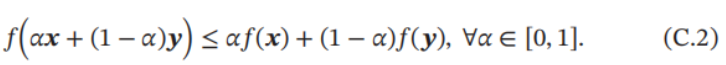凸优化问题是一种特殊的约束优化问题，需满足目标函数为凸函数，并且等式约束函数为线性函数，不等式约束函数为凸函数．

## 2. 优化算法

优化问题一般都可以通过迭代的方式来求解：通过猜测一个初始的估计𝒙0，然后不断迭代产生新的估计 𝒙1 , 𝒙2 , ⋯ 𝒙𝑡，希望 𝒙𝑡 最终收敛到期望的最优解 𝒙∗．

一个好的优化算法应该是在一定的时间或空间复杂度下能够快速准确地找到最优解．同时，好的优化算法受初始猜测点的影响较小，通过迭代能稳定地找到最优解𝒙∗ 的邻域，然后迅速收敛于𝒙∗．

优化算法中常用的迭代方法有线性搜索和置信域方法等．线性搜索的策略是寻找方向和步长，具体算法有梯度下降法、牛顿法、共轭梯度法等．

### 2.1 全局最小解和局部最小解

对于很多非线性优化问题，会存在若干个局部最小值（Local Minima），其对应的解称为局部最小解（Local Minimizer）． 局部最小解𝒙∗ 定义为：存在一个𝛿 > 0，对于所有的满足‖𝒙 − 𝒙∗‖ ≤ 𝛿 的𝒙，都有 𝑓(𝒙∗ ) ≤ 𝑓(𝒙)．也就是说，在𝒙∗的邻域内，所有的函数值都大于或者等于𝑓(𝒙∗)．对于所有的 𝒙 ∈ 𝒟，都有 𝑓(𝒙∗ ) ≤ 𝑓(𝒙) 成立，则 𝒙∗ 为全局最小解（Global Minimizer）．

求局部最小解一般是比较容易的，但很难保证其为全局最小解．对于线性规划或凸优化问题，局部最小解就是全局最小解．

要确认一个点 𝒙∗ 是否为局部最小解，通过比较它的邻域内有没有更小的函数值是不现实的．如果函数𝑓(𝒙)是二次连续可微的，我们可以通过检查目标函数在点𝒙∗ 的梯度∇𝑓(𝒙∗)和Hessian矩阵∇2𝑓(𝒙∗)来判断．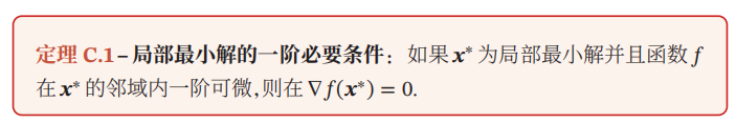证明*.* 如果函数 𝑓(𝒙) 是连续可微的，根据泰勒公式（Taylor’s Formula），函数𝑓(𝒙)的一阶展开可以近似为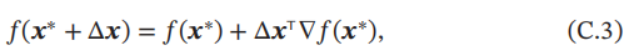假设∇𝑓(𝒙∗ ) ≠ 0，则可以找到一个 Δ𝒙（比如 Δ𝒙 = −𝛼∇𝑓(𝒙∗)，𝛼 为很小的正数），使得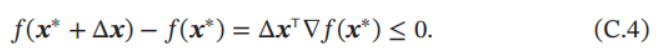这和局部最小的定义矛盾．

函数 𝑓(𝒙) 的一阶偏导数为 0 的点也称为驻点（Stationary Point）或临界点（Critical Point）．驻点不一定为局部最小解．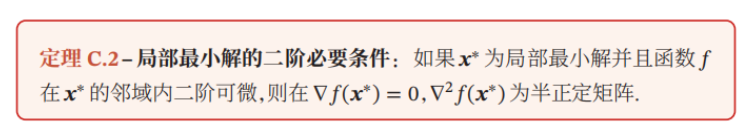证明*.* 如果函数𝑓(𝒙)是二次连续可微的，函数𝑓(𝒙)的二阶展开可以近似为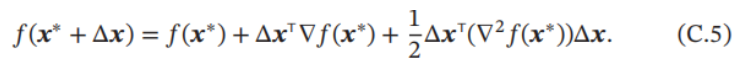由一阶必要性定理可知∇𝑓(𝒙∗ ) = 0，则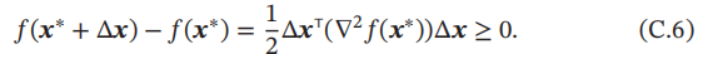即∇2𝑓(𝒙∗)为半正定矩阵．

### 2.2 梯度下降法

对于函数𝑓(𝒙)，如果𝑓(𝒙)在点𝒙𝑡 附近是连续可微的，那么𝑓(𝒙)下降最快的方向是 𝑓(𝒙)在𝒙𝑡 点的梯度方法的反方向．

根据泰勒一阶展开公式，有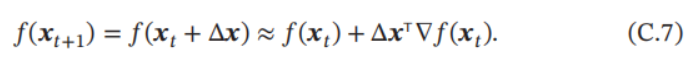要使得 𝑓(𝒙𝑡+1) < 𝑓(𝒙𝑡)，就得使 Δ𝒙T∇𝑓(𝒙𝑡 ) < 0．我们取Δ𝒙 = −𝛼∇𝑓(𝒙𝑡)．如果𝛼 > 0为一个够小数值时，那么 𝑓(𝒙𝑡+1) < 𝑓(𝒙𝑡)成立．

这样我们就可以从一个初始值𝒙0 出发，通过迭代公式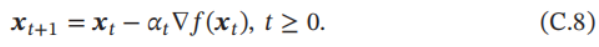生成序列 𝒙0 , 𝒙1 , 𝒙2, ⋯ 使得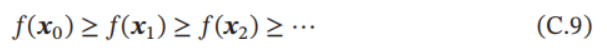如果顺利的话，序列 (𝒙𝑛)收敛到局部最小解𝒙∗．注意，每次迭代步长 𝛼 可以改变，但其取值必须合适，如果过大就不会收敛，如果过小则收敛速度太慢．梯度下降法的过程如图C.1所示．曲线是等高线（水平集），即函数𝑓 为不同常数的集合构成的曲线．红色的箭头指向该点梯度的反方向（梯度方向与通过该点的等高线垂直）．沿着梯度下降方向，将最终到达函数𝑓 值的局部最小解．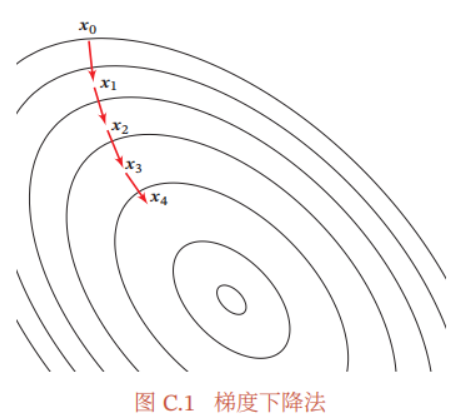## 3. 拉格朗日乘数法与KKT条件

拉格朗日乘数法（Lagrange Multiplier） 是一种有效求解约束优化问题的优化方法．

约束优化问题可以表示为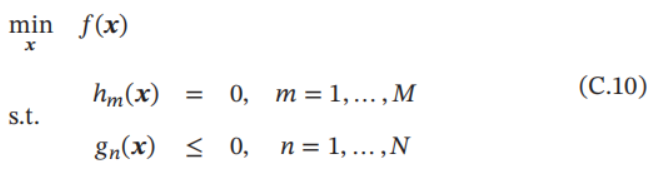其中ℎ𝑚(𝒙)为等式约束函数，𝑔𝑛(𝒙)为不等式约束函数．𝒙的可行域为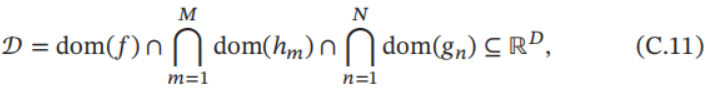其中dom(𝑓)是函数𝑓 的定义域．

### 3.1 等式约束优化问题

如果公式(C.10)中只有等式约束，我们可以构造一个拉格朗日函数Λ(𝒙, 𝜆)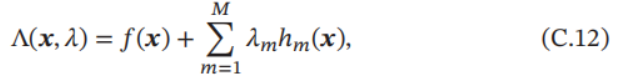其中 𝜆 为拉格朗日乘数，可以是正数或负数．如果 𝑓(𝒙∗) 是原始约束优化问题的局部最优值，那么存在一个𝜆∗ 使得(𝒙∗ , 𝜆∗)为拉格朗日函数Λ(𝒙, 𝜆)的驻点．因此，只需要令 𝜕Λ(𝒙,𝜆)/𝜕𝒙= 0和 𝜕Λ(𝒙,𝜆)/𝜕𝜆= 0，得到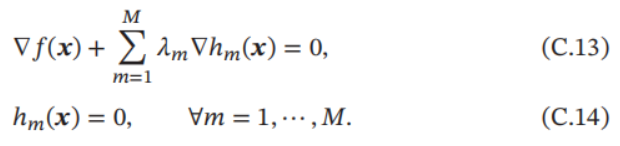上面方程组的解即为原始问题的可能解．因为驻点不一定是最小解，所以在实际应用中需根据具体问题来验证是否为最小解．

拉格朗日乘数法是将一个有𝐷个变量和𝑀 个等式约束条件的最优化问题转换为一个有 𝐷 + 𝑀 个变量的函数求驻点的问题．拉格朗日乘数法所得的驻点会包含原问题的所有最小解，但并不保证每个驻点都是原问题的最小解．

### 3.2 不等式约束优化问题

对于公式(C.10)中定义的一般约束优化问题，其拉格朗日函数为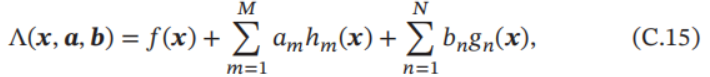其中𝒂 = [𝑎1 , ⋯ , 𝑎𝑀]T 为等式约束的拉格朗日乘数，𝒃 = [𝑏1 , ⋯ , 𝑏𝑁 ]T 为不等式约束的拉格朗日乘数．

当约束条件不满足时，有 max𝒂,𝒃 Λ(𝒙, 𝒂, 𝒃) = ∞；当约束条件满足时并且𝒃 ≥ 0时，max𝒂,𝒃 Λ(𝒙, 𝒂, 𝒃) = 𝑓(𝒙)．因此，原始约束优化问题等价于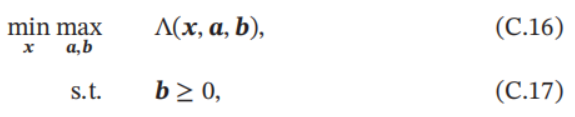这个min-max优化问题称为主问题（Primal Problem）．

对偶问题 主问题的优化一般比较困难，我们可以通过交换 min-max 的顺序来简化．定义拉格朗日对偶函数为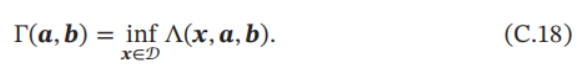Γ(𝒂, 𝒃)是一个凹函数，即使𝑓(𝒙)是非凸的． 当𝒃 ≥ 0时，对于任意的 ̃ 𝒙 ∈ 𝒟，有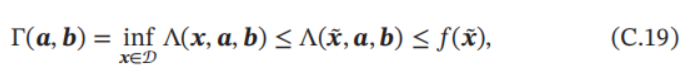令𝒑∗ 是原问题的最优值，则有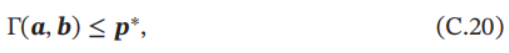即拉格朗日对偶函数Γ(𝒂, 𝒃)为原问题最优值的下界．

优化拉格朗日对偶函数 Γ(𝒂, 𝒃) 并得到原问题的最优下界，称为拉格朗日对偶问题（Lagrange Dual Problem）．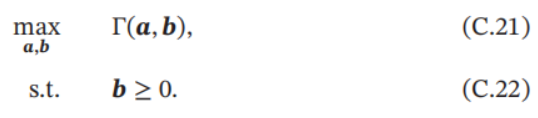拉格朗日对偶函数为凹函数，因此拉格朗日对偶问题为凸优化问题．

令𝒅∗ 表示拉格朗日对偶问题的最优值，则有𝒅∗ ≤ 𝒑∗，这个性质称为弱对偶性（Weak Duality）．如果𝒅∗ = 𝒑∗，这个性质称为强对偶性（Strong Duality）．

当强对偶性成立时，令𝒙∗ 和𝒂∗, 𝒃∗ 分别是原问题和对偶问题的最优解，那么它们满足以下条件：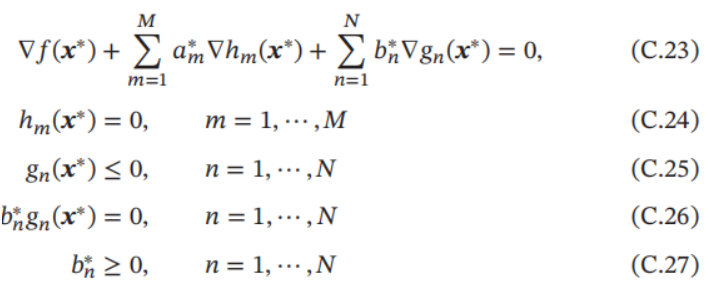这 5 个条件称为不等式约束优化问题的KKT 条件（Karush-Kuhn-Tucker Condition）．KKT 条件是拉格朗日乘数法在不等式约束优化问题上的泛化．当原问题是凸优化问题时，满足KKT条件的解也是原问题和对偶问题的最优解． 在KKT条件中，需要关注的是公式(C.26)，称为互补松弛（ComplementarySlackness）条件．如果最优解 𝒙∗ 出现在不等式约束的边界上 𝑔𝑛(𝒙) = 0，则𝑏∗𝑛 > 0；如果最优解𝒙∗ 出现在不等式约束的内部𝑔𝑛(𝒙) < 0，则𝑏∗𝑛 = 0．互补松弛条件说明当最优解出现在不等式约束的内部，则约束失效．

展开全文算法 机器学习 聚类 人工智能
• 本篇文章是对约瑟夫环的数学优化方法进行了详细的分析介绍，需要的朋友参考下
•文档
• 利用模糊数学优化VAR风险指标的投资组合，孟宇，支晓云，本文引入目前流行的风险指标VAR，以收益率与风险损失为目标，将模糊数学运用于有价证券组合选择，按投资者给定的期望目标及容差，
• 数学优化方案高中数学空间向量与立体几何苏教选修PPT学习教案.pptx
•学习课件
• ## 数学优化入门：凸优化

万次阅读 多人点赞 2016-10-18 11:10:50
做科研时，曾花了段时间学习凸优化，后来发现ML中其应用也非常普遍，想来今后可能还会接触，干脆做个系统的...凸优化，是数学优化的一个子领域，研究定义于凸集中的凸函数最小化的问题。虽然条件苛刻，但应用广泛...

做科研时，曾花了段时间学习凸优化，后来发现ML中其应用也非常普遍，想来今后可能还会接触，干脆做个系统的总结，方便以后查询。

博文内容主要参考Boyd（Stanford）的Convex Optimization，配套的slides，以及部分网络材料，感兴趣的朋友可以一起学习探讨。

# 1、前言

凸优化，是数学最优化的一个子领域，研究定义于凸集中的凸函数最小化的问题。虽然条件苛刻，但应用广泛，具有重要价值，主要体现在：

• 凸优化本身具有很好的性质
一来，凸问题的局部最优解就是全局最优解。二来，凸优化理论中的Lagrange对偶，为凸优化算法的最优性与有效性提供了保证。近些年来关于凸问题的研究非常透彻，以至于只要把某一问题抽象为凸问题，就可以近似认为这个问题已经解决了。

• 凸优化具有很强扩展性
对于非凸问题，通过一定的手段，要么可以等价地化归为凸问题，要么可以用凸问题去近似、逼近得到边界。例如，几何规划、整数规划，虽然本身是非凸的，但是可以借助凸优化手段去解，这就极大地扩张了凸优化的应用范围。
以深度学习来说，其中关键的反向传播（Back Propagation）算法，本质就是凸优化算法中的梯度下降法，即使问题极度非凸，梯度下降还是有很好的表现，当然深度学习的机制还有待研究。

• 凸优化的应用十分广泛
如线性回归、范数逼近、插值拟合、参数估计，以及许多的几何问题等。

• 针对其他非凸问题的研究还不充分
凸优化之重要，从另一个角度说，就是我们没有找到很好的非凸优化的算法，这一部分还有许多学者都在努力。

虽然说凸优化的研究已经比较成熟，但由于还没有行业公认的通行解决方法，所以Boyd也说过“we cannot claim that solving general convex optimization problem is a technology, like solving least-squares or linear programming problems……it is fair to say that interior-point methods are approaching a technology”，即目前已有的凸优化方法还不能称之为技术。但还是说那句话，基本上，如果你把一个现实问题建成凸优化问题模型，你就可以认为这个问题已经被解决了。

多说几句，对于非凸优化中，凸优化同样起到很重要的作用：

• 解决一个非凸优化问题时，可以先试图建立一个简化的多凸优化模型，解出以后作为非凸问题的一个起始点

• 很多非凸优化问题的启发式算法的基础都是基于凸优化

• 可以先建立非凸优化的松弛问题，使用凸优化算法求解，然后作为非凸优化问题的上限或下限

那么，下面将分几章花些篇幅好好讲解下凸优化的主要知识点和常用结论。

# 2、凸集

## 2.1 定义

A set C C $C$ is convex, if $\theta x+\left(1-\theta \right)y\in C$$\theta x + (1-\theta)y \in C$, for any x,yC x , y ∈ C $x,y \in C$ and θR θ ∈ R $\theta \in R$ with 0θ1 0 ≤ θ ≤ 1 $0 \le \theta \le 1$.

简言之，凸集即过集合C内任意两点的线段均在集合C内。

## 2.2 常用凸集

• Rn R n $R^n$
• The non-negative orthant: Rn+ R + n $R_+^n$
• Norm ball: {x:x1} { x : ‖ x ‖ ≤ 1 } $\{x: \|x\| \le 1 \}$
• Affine subspace: {xRn:Ax=b,ARn×n,bRn×1} { x ∈ R n : A x = b , A ∈ R n × n , b ∈ R n × 1 } $\{ x \in R^n: Ax=b,A \in R^{n \times n},b \in R^{n \times 1} \}$
• Polyhedra: {xRn:Ax=b} { x ∈ R n : A x ≺= b } $\{ x \in R^n: Ax \prec = b\}$
• Intersections of convex sets (Note here that the union of convex sets in general is not convex)
• Postive semidefinite cone: Sn+={XSn|X=0} S + n = { X ∈ S n | X ≻= 0 } $S_+^n=\{ X \in S^n | X \succ = 0 \}$ (Note: 将前述半正定矩阵改为正定阵、负定阵、半负定阵，仍成立)

# 3、凸函数

## 3.1 定义

A function f:RnR f : R n → R $f:R^n \to R$ is convex, if its domain D(f) D ( f ) $D(f)$ is a convex set, and if f(θx+(1θ)y)θf(x)+(1θ)f(y) f ( θ x + ( 1 − θ ) y ) ≤ θ f ( x ) + ( 1 − θ ) f ( y ) $f(\theta x + (1-\theta)y) \le \theta f(x) + (1-\theta)f(y)$ for all x,yD(f) x , y ∈ D ( f ) $x,y \in D(f)$ and θR,0θ1 θ ∈ R , 0 ≤ θ ≤ 1 $\theta \in R, 0 \le \theta \le 1$.

凸函数的一阶微分条件

Suppose a function f:RnR f : R n → R $f:R^n \to R$ is differentiable, then f f $f$ is convex if and only if: $D\left(f\right)$$D(f)$ is a convex set and for all x,yD(f) x , y ∈ D ( f ) $x,y \in D(f)$, f(y)f(x)+(xf(x))T(yx) f ( y ) ≥ f ( x ) + ( ∇ x f ( x ) ) T ( y − x ) $f(y) \ge f(x) + (\nabla_xf(x))^T(y-x)$.

凸函数的二阶微分条件

Suppose a function f:RnR f : R n → R $f:R^n \to R$ is twice differentiable, then f f $f$ is convex if and only if: $D\left(f\right)$$D(f)$ is a convex set and its Hessian is postive semidefinite.

## 3.2 常用凸函数

• 负熵函数： xlogx x l o g x $xlogx$
• 范数函数： xp ‖ x ‖ p $\| x \|_p$
• f(x)=max(x1,...,xn) f ( x ) = m a x ( x 1 , . . . , x n ) $f(x)=max(x_1,...,x_n)$
• f(x)=log(ex1+...+exn) f ( x ) = l o g ( e x 1 + . . . + e x n ) $f(x)=log(e^{x_1}+...+e^{x_n})$
• f(x)=(ni=1xi)1/n,D(f)=Rn++ f ( x ) = ( ∏ i = 1 n x i ) 1 / n , D ( f ) = R + + n $f(x)=(\prod\nolimits_{i = 1}^n {{x_i}} )^{1/n}, D(f)=R_{++}^n$
• f(X)=log(detX),D(f)=Sn++ f ( X ) = l o g ( det X ) , D ( f ) = S + + n $f(X)=log(\text{det}X), D(f)=S_{++}^n$

## 3.3 保凸运算

• 凸函数的非负加权和
• 凸函数与仿射变换的复合： g(x)=f(Ax+b) g ( x ) = f ( A x + b ) $g(x)=f(Ax+b)$
• 逐点最大、最小值： f(x)=max(f1(x),...,fn(x)) f ( x ) = m a x ( f 1 ( x ) , . . . , f n ( x ) ) $f(x)=max(f_1(x),...,f_n(x))$ g(x)=infyCf(x,y) g ( x ) = i n f y ∈ C f ( x , y ) $g(x)=inf_{y \in C} f(x,y)$
• 透视变换： g(x,t)=tf(x/t) g ( x , t ) = t f ( x / t ) $g(x,t)=tf(x/t)$

# 4、凸优化问题

## 4.1 定义

通常将一个优化问题写成以下标准形式：

mins.t.:f(x)gi(x)0,i=1,...,mhj(x)=0,j=1,...,t min f ( x ) s . t . : g i ( x ) ≤ 0 , i = 1 , . . . , m h j ( x ) = 0 , j = 1 , . . . , t

f(x) f ( x ) $f(x)$ gi(x) g i ( x ) $g_i(x)$均为凸函数， hj(x) h j ( x ) $h_j(x)$均为仿射函数时，上述优化问题称之为凸优化问题。

关于凸优化问题再补充几个特点：

• 一般没有解析解
• 不考虑等式约束时，计算复杂度大致正比于 max{n3,n2m,F} max { n 3 , n 2 m , F } $\max \left\{ {{n^3},{n^2}m,F} \right\}$，其中 F F $F$是对所有${g}_{i}$$g_i$及其一阶、二阶导的计算代价
• 虽然难以识别，但可以通过很多手段进行转化

## 4.2 优化问题的等价形式

注意以下等价形式不要求为凸问题，等价和相同不是一个概念。

• 变量替换
• 目标函数和约束函数的变换
• 松弛变量（不等式约束转变为等式约束加非负约束）
• 消除等式约束
• 消除线性等式约束
• 引入等式约束
• 优化部分变量
• 上境图问题形式
• 隐式与显式约束

## 4.3 常用凸优化问题

### 1）Least-squares（LS）

作为凸优化问题的一个特例，其成熟解法已经可以称之为technology。

对于Least-squares问题： minAxb22 min ‖ A x − b ‖ 2 2 $\min \left\| {Ax - b} \right\|_2^2$，其解析解为 x=(ATA)1ATb x ∗ = ( A T A ) − 1 A T b ${x^*} = {\left( {{A^T}A} \right)^{ - 1}}{A^T}b$，对于 Ak×n A ∈ R k × n $A \in {\mathbb{R}^{k \times n}}$，在不结构化情况下，计算复杂度为 O(n2k) O ( n 2 k ) ${\rm O}\left( {{n^2}k} \right)$

### 2）Linear programming（LP）

作为凸优化问题的一个特例，虽然没有解析解，但仍有可靠高效的算法和工具解决，也可以称之为technology。

对于Linear programming问题：

mins.t.:cTx+daTixbi,i=1,...,m min c T x + d s . t . : a i T x ≤ b i , i = 1 , . . . , m

mn m ≥ n $m \ge n$时，计算复杂度为 O(n2m) O ( n 2 m ) ${\rm O}\left( {{n^2}m} \right)$

但线性规划问题并不像前面的最小二乘问题那么好辨别，通常需要通过一些标准的小技巧将原问题转化为线性规划问题，如包含 l1 l 1 $l_1$范数或者 l l ∞ $l_\infty$范数，或者分段线性函数的问题等。

mins.t.:12xTPx+qTx+rGx=hAx=bPSn+ min 1 2 x T P x + q T x + r s . t . : G x ≺= h A x = b P ∈ S + n

mins.t.:12xTP0x+qT0x+r012xTPix+qTix+riAx=bPiSn+ min 1 2 x T P 0 x + q 0 T x + r 0 s . t . : 1 2 x T P i x + q i T x + r i A x = b P i ∈ S + n

### 5）Second-order cone programming（SOCP）

mins.t.:fTxAix+b2cTix+diFx=g min f T x s . t . : ‖ A i x + b ‖ 2 ≤ c i T x + d i F x = g

### 6）Semidefinite programming （SDP）

mins.t.:Tr(CX)Tr(AiX)=biX=0 min T r ( C X ) s . t . : T r ( A i X ) = b i X ≻= 0

# 5、Lagrange Duality

对于有约束的优化问题，通过拉格朗日法可以将其转变为等价的无约束优化问题。在这个过程中，新构造的拉格朗日函数存在好玩的对偶性质，从而衍生出了对偶问题。原问题与对偶问题之间的特殊性质，为我们研究优化问题提供了新的方向和方法。

因此，这部分的思路是：对4.1定义的优化问题，通过拉格朗日法构造拉格朗日函数，从而生成原问题Primal problem和对偶问题Dual problem，然后介绍一些引理，揭示原问题与对偶问题之间的关系。

## 5.1 Primal problem

首先要求4.1提出的标准优化问题中的 f(x) f ( x ) $f(x)$ gi(x) g i ( x ) $g_i(x)$ hj(x) h j ( x ) $h_j(x)$均为连续可微函数，构造广义拉格朗日函数

L(x;α,β)=f(x)+i=1mαigi(x)+j=1tβjhj(x) L ( x ; α , β ) = f ( x ) + ∑ i = 1 m α i g i ( x ) + ∑ j = 1 t β j h j ( x )

其中， α α $\alpha$ β β $\beta$是拉格朗日乘子，且 αi0 α i ≥ 0 $\alpha_i \ge 0$ x x $x$称为primal变量，$\alpha$$\alpha$ β β $\beta$称为dual变量。
注意， L(x;α,β) L ( x ; α , β ) $L(x;\alpha,\beta)$ 是关于 α α $\alpha$ β β $\beta$ 的仿射函数。

从而，我们可以构造Primal problem

minxθP(x)=minx[maxα,β;αi0L(x;α,β)] min x ⁡ θ P ( x ) = min x ⁡ [ max α , β ; α i ≥ 0 ⁡ L ( x ; α , β ) ]

显然有：

θP(x)=f(x)+{0,ifxisprimalfeasible+,ifxisnotprimalfeasible θ P ( x ) = f ( x ) + { 0 , i f x i s p r i m a l f e a s i b l e + ∞ , i f x i s n o t p r i m a l f e a s i b l e

其中，primal feasible意味着 x x $x$满足4.1优化问题中的所有约束条件。
注意，${\theta }_{P}\left(x\right)$${\theta _P}(x)$是关于 x x $x$的凸函数，因此，主问题求解的核心在内层最大化。

因此，可以看出这里构建的primal problem和4.1的优化问题是等价的，即同解。这样一来，就把原始优化问题表示成了广义拉格朗日函数的极小极大问题，我们定义primal problem的最优值${p}^{\ast }=\underset{x}{min}{\theta }_{P}\left(x\right)={\theta }_{P}\left({x}^{\ast }\right)$$p^*=\mathop {\min }\limits_x {\theta _P}(x)={\theta _P}(x^*)$，称为primal problem的值。

## 5.2 Dual problem

好了，下面我们来构造神奇的Dual problem

maxα,β;αi0θD(α,β)=maxα,β;αi0[minxL(x;α,β)] max α , β ; α i ≥ 0 ⁡ θ D ( α , β ) = max α , β ; α i ≥ 0 ⁡ [ min x ⁡ L ( x ; α , β ) ]

对于上述广义拉格朗日函数的极大极小问题，定义dual problem的最优值 d=minα,β;αi0θD(α,β)=θD(α,β) d ∗ = min α , β ; α i ≥ 0 ⁡ θ D ( α , β ) = θ D ( α ∗ , β ∗ ) $d^*=\mathop {\min }\limits_{\scriptstyle\alpha ,\beta; {\alpha _i} \ge 0} {\theta _D}(\alpha,\beta) ={\theta _D}(\alpha^*,\beta^*)$，称为primal problem的值。
注意， θD(α,β) θ D ( α , β ) ${\theta _D}(\alpha,\beta)$是关于 α α $\alpha$ β β $\beta$的凹函数，因此，对偶问题求解的核心在内层最小化。。

## 5.3 Primal problem与Dual problem的关系

### 1）Lemma 1

If (α,β) ( α , β ) $(\alpha, \beta)$ are dual feasible, then θD(α,β)p θ D ( α , β ) ≤ p ∗ ${\theta _D}(\alpha,\beta) \le p^*$.

### 2）Lemma 2（Weak Duality）

For any pair of primal and dual problems, dp d ∗ ≤ p ∗ $d^* \le p^*$.

注意，此性质always holds，无论优化问题是凸的还是非凸的，通常用来寻找困难问题的下界。

### 3）Lemma 3 （Strong Duality）

For any pair of primal and dual problems, which satisfy certain conditions called constraint qualifications, then d=p d ∗ = p ∗ $d^* = p^*$.

实际上有不少constraint qualifications可以保证强对偶，我们介绍几种常用的constraint qualification：

• f=f f = f ∗ ∗ $f=f^{{**}}$ where f f $f$ is the perturbation function relating the primal and dual problems and $f={f}^{\ast \ast }$$f=f^{{**}}$ is the biconjugate of f f $f$

• $f$$f$ is convex and lower semi-continuous (equivalent to the first point by the Fenchel-Moreau theorem)

• the primal problem is a linear OP

• Slater’s condition for a convex optimization problem

其中，Slater’s condition更常用，we say that the problem satisfies Slater’s condition if it is strictly feasible, that is:

x0D(f):gi(x0)<0,i=1,...,m;hj(x0)=0,j=1,...,t ∃ x 0 ∈ D ( f ) : g i ( x 0 ) < 0 , i = 1 , . . . , m ; h j ( x 0 ) = 0 , j = 1 , . . . , t

即，if the primal problem is convex, and satisfies the weak Slater’s condition, then strong duality holds.

注意，有些非凸问题也可以满足强对偶。

## 5.4 Complementary slackness

也称作KKT complementarity，即

If strong duality holds, then αigi(x)=0 α i ∗ g i ( x ∗ ) = 0 $\alpha _i^*{g_i}\left( {{x^*}} \right) = 0$ for any i i $i$.

直观来看，就是如果${\alpha }_{i}^{\ast }>0$$\alpha _i^*>0$ gi(x)=0 g i ( x ∗ ) = 0 ${g_i}\left( {{x^*}} \right) = 0$，如果 gi(x)<0 g i ( x ∗ ) < 0 ${g_i}\left( {{x^*}} \right)<0$ αi=0 α i ∗ = 0 $\alpha _i^*=0$

## 5.5 KKT条件

有以下逻辑：当strong duality存在时； x x ∗ $x^*$为primal optiaml和 α,β α ∗ , β ∗ $\alpha^*,\beta^*$为dual optiaml，则KKT条件成立；当KKT条件成立，则 x x ∗ $x^*$为primal optiaml和 α,β α ∗ , β ∗ $\alpha^*,\beta^*$为dual optiaml。

下面介绍KKT条件：

xisprimalfeasibleα,βisdualfeasiblecomplementaryslacknessxL(x;α,β)=0 { x ∗ i s p r i m a l f e a s i b l e α ∗ , β ∗ i s d u a l f e a s i b l e c o m p l e m e n t a r y s l a c k n e s s ∇ x L ( x ∗ ; α ∗ , β ∗ ) = 0

## 5.6 常用案例

1） 如果 f(x),gi(x) f ( x ) , g i ( x ) $f(x),g_i(x)$是凸函数， hj(x) h j ( x ) $h_j(x)$是仿射函数，且存在 x x $x$使${g}_{i}\left(x\right)<0$$g_i(x)<0$对多有 i i $i$成立，则存在${x}^{\ast };{\alpha }^{\ast },{\beta }^{\ast }$${{x^*};{\alpha ^*},{\beta ^*}}$使得 x x ∗ $x^*$是原始问题的解， α,β α ∗ , β ∗ ${\alpha ^*},{\beta ^*}$是对偶问题的解，且 p=d=L(x;α,β) p ∗ = d ∗ = L ( x ∗ ; α ∗ , β ∗ ) $p^*=d^*=L\left( {{x^*};{\alpha ^*},{\beta ^*}}\right)$

2） 如果 f(x),gi(x) f ( x ) , g i ( x ) $f(x),g_i(x)$是凸函数， hj(x) h j ( x ) $h_j(x)$是仿射函数，且存在 x x $x$使${g}_{i}\left(x\right)<0$$g_i(x)<0$对多有 i i $i$成立，则“${x}^{\ast }$$x^*$是原始问题的解， α,β α ∗ , β ∗ ${\alpha ^*},{\beta ^*}$是对偶问题的解”与“ x;α,β x ∗ ; α ∗ , β ∗ ${{x^*};{\alpha ^*},{\beta ^*}}$满足KKT条件”是充要关系。

展开全文• JuMPTutorials.jl：有关在Julia中使用JuMP进行数学优化的教程
• 北师大小学四年级数学优化PPT教案学习.pptx
• 数学高高三数学优化设计第二轮复习专题数列求和及其综合应用PPT学习教案.pptx
• ensmallen提供了一组简单的抽象，用于编写目标函数进行优化。 它还提供了大量标准和凸优化器，几乎可用于任何数学优化任务。 这些包括完整批次梯度下降技术，小批量技术，无梯度优化和约束优化。
• O'Reilly数学优化入门 O'Reilly在线培训“数学优化入门”的材料Python
• 凸优化学习------数学优化问题的分类 一、 无约束优化和有约束优化        在连续优化问题中，根据是否有变量的约束条件，可以将优化问题分为无约束优化问题和约束优化问题。有...

# 凸优化学习------数学优化问题的分类

#### 一、 无约束优化和有约束优化

在连续优化问题中，根据是否有变量的约束条件，可以将优化问题分为无约束优化问题和约束优化问题。有变量的约束条件则为约束优化问题，反之则为无约束优化问题。

#### 二、 线性优化和非线性优化

目标函数和所有的约束函数都为线性函数，则该问题为线性规划问题（Linear Programming）。相反，如果目标函数或任何一个约束函数为非线性函数，则该问题为非线性规划问题（Nonlinear Programming）
在非线性优化问题中，有一类比较特殊的问题是凸优化问题（Convex Programming）。在凸优化问题中，变量 x 的可行域为凸集，即对于集合中任意两点，它们的连线全部位于在集合内部。目标函数 f 也必须为凸函数，即满足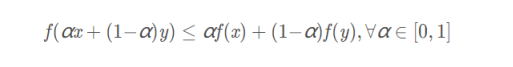凸优化问题是一种特殊的约束优化问题，需满足目标函数为凸函数，并且等式约束函数为线性函数，不等式约束函数为凹函数。

#### 三、 连续优化和离散优化

根据输入变量 x 的值域是否为实数域，数学优化问题可以分为离散优化问题和连续优化问题。

###### 3.1 连续优化问题

连续优化（Continuous Optimization问题是目标函数的输入变量为连续变量 x ∈ Rd，即目标标函数为实函数。

###### 3.2 离散优化问题

3.2.1 组合优化（Combinatorial Optimization）：
其目标是从一个有限集合中找出使得目标函数最优的元素。在一般的组合优化问题中，集合中的元素之间存在一定的关联，可以表示为图结构。典型的组合优化问题有旅行商问题、最小生成树问题、图着色问题等。很多机器学习问题都是组合优化问题，比如特征选择、聚类问题、超参数优化问题以及**结构化学习（Structured Learning）**中标签预测问题等。
3.2.2 整数优化
输入变量 x ∈ Zd为整数。一般常见的整数规划问题为整数线性规划（Integer Linear Programming，ILP）。整数线性规划的一种最直接的求解方法是：（1）去掉输入必须为整数的限制，将原问题转换为一般的线性规划问题，这个线性规划问题为原问题的松弛问题；（2）求得相应松弛问题的解；（3）把松弛问题的解四舍五入到最接近的整数。但是这种方法得到的解一般都不是最优的，因此原问题的最优解不一定在松弛问题最优解的附近。另外，这种方法得到的解也不一定满足约束条件。

#### 四、 单目标优化和多目标优化

###### 4.1 单目标优化（Single-Objective Optimization）

所评测目标只有一个，只需要根据具体的满足函数条件，求得最值

###### 4.2 多目标优化（Multi-objective Optimization）

多个评测函数的存在，而且使用不同的评测函数的解，也是不同的。也即是说：多目标优化问题中，同时存在多个最大化或是最小化的目标函数，并且，这些目标函数并不是相互独立的，也不是相互和谐融洽的，他们之间会存在或多或少的冲突，使得不能同时满足所有的目标函数。一般情况下多目标优化情况并没有唯一的全局最优解

#### 五、 静态规划和动态规划

###### 5.1 静态规划（Static Programming）

静态规划简单来说是穷举事件所有解决方法，从中选出最优的一个解。静态规划最大的特点是时间复杂度特别高，当问题规划很大时计算量是一个天文数字。同时这种方法也有优点，就是穷举过程中我们始终存在着一个可行解。这种方法，从一个或多个可行解开始，通过不断迭代对现有可行解优化，最后得到最优解。这种方法称为静态规划。

###### 5.2 动态规划（Dynamic Programming）

动态规划是运筹学的一个分支，是求解决策过程最优化的过程。是通过比较当前决策状态和未来几个状态的比较进行选取最优，关键点是找到状态转移方程。

###### 5.2.1 动态规划概念引入：

有一类活动的过程，由于它的特殊性，可将过程分成若干个互相联系的阶段，在它的每一阶段都需要做决策，从而使整个过程达到最好的活动效果。因此各个阶段决策的选取不能任意确定，它依赖于当前面临的状态，又影响以后的发展。当各个阶段决策确定后，就组成一个决策序列，因而也就确定了整个过程的一条活动路线．这种把一个问题看作是一个前后关联具有链状结构的多阶段过程就称为多阶段决策过程，这种问题称为多阶段决策问题。在多阶段决策问题中，各个阶段采取的决策，一般来说是与时间有关的，决策依赖于当前状态，又随即引起状态的转移，一个决策序列就是在变化的状态中产生出来的，故有“动态”的含义，称这种解决多阶段决策最优化的过程为动态规划方法。

###### 5.2.2 动态规划基本思想

动态规划算法通常用于求解具有某种最优性质的问题。在这类问题中，可能会有许多可行解。每一个解都对应于一个值，我们希望找到具有最优值的解。动态规划算法与分治法类似，其基本思想也是将待求解问题分解成若干个子问题，先求解子问题，然后从这些子问题的解得到原问题的解。与分治法不同的是，适合于用动态规划求解的问题，经分解得到子问题往往不是互相独立的。若用分治法来解这类问题，则分解得到的子问题数目太多，有些子问题被重复计算了很多次。如果我们能够保存已解决的子问题的答案，而在需要时再找出已求得的答案，这样就可以避免大量的重复计算，节省时间。我们可以用一个表来记录所有已解的子问题的答案。不管该子问题以后是否被用到，只要它被计算过，就将其结果填入表中。这就是动态规划法的基本思路。具体的动态规划算法多种多样，但它们具有相同的填表格式。

###### 5.2.3 动态规划局限性：

动态规划对于解决多阶段决策问题的效果是明显的，但是动态规划也有一定的局限性。首先，它没有统一的处理方法，必须根据问题的各种性质并结合一定的技巧来处理；另外当变量的维数增大时，总的计算量及存贮量急剧增大。因而，受计算机的存贮量及计算速度的限制，当今的计算机仍不能用动态规划方法来解决较大规模的问题，这就是**“维数障碍”**。

#### 六、 随机规划

随机规划是规划论的一个分支，线性规划的推广。研究约束条件中的系数和目标函数中的参数均为随机变量时的线性规划问题。用于研究具有不确定性的决策问题。随机规划的中心问题是选择参数，使收益的数学期望达到最大，或使成本的数学期望达到极小。根据数学模型求得问题的最优解，但这个最优解一般不是一个确定值而是一个期望值。在随机规划中，需对随机变量进行描述，分析其概率分布，往往还要考虑各随机变量的自相关和互相关，因而在理论上和求解方法上都比确定性规划复杂得多。实际上，求解随机规划问题时，总是设法把它转化成确定性数学规划问题，再进行求解。如果随机变量的非确定性或者量的变化很小，对系统的性能不产生严重影响，可以用其数学期望代替这个非确定值，并用确定性方法求解；然后通过敏感性分析来估计非确定性因素对方案的影响程度。如果随机变量变化很大，用期望值可能使方案性能的评价受到很大影响，这时就要用随机规划方法求解。
常见的随即规划问题：报童问题。
常用方法：样本均值方法。

#### 七、 鲁棒规划

###### 7.1 鲁棒优化定义

鲁棒优化的目的是求得这样一个解，对于可能出现的所有情况，约束条件均满足，并且使得最坏情况下的目标函数的函数值最优。 其核心思想是将原始问题以一定的近似程度转化为一个具有多项式计算复杂度的凸优化问题。鲁棒优化的关键是建立相应的鲁棒对等模型。然后利用相关的优化理论将其转化为可求解的“近似”鲁棒对等问题，并给出鲁棒最优解。

###### 7.2 鲁棒优化原理

鲁棒优化与其它不确定优化方法的最大区别在于：
①鲁棒优化强调的是所谓的硬约束，寻求一个对于不确定输入的所有实现都能有良好性能的解，即不确定优化问题的解对于任何一个可能参数的实现都必须是可行的，而其它不确定优化问题并没有这个要求。
②鲁棒优化的建模思想与其它优化方法不同，它是以最坏情况下的优化为基础，这代表了一个保守的观点。得到的优化方案并不是最优的，但是，当参数在给定的集合内发生变化时，仍能确保优化方案是可行的，使模型具有一定的鲁棒性,即优化方案对参数扰动不敏感。
③鲁棒优化对于不确定参数没有分布假定，只是给出不确定参数集，不确定参数集合内的所有值都同等重要

###### 7.3 鲁棒优化适用范围

鲁棒优化是解决内部结构和外部环境不确定环境下的一种新的优化方法。鲁棒优化解决内部结构变动问题时,主要是约束条件参数的不确定性或目标函数参数的不确定性解决外部环境变化时，主要是外界不确定性扰动。鲁棒优化己经从最初的线性优化鲁棒方法，发展到鲁棒优化理论的经典体系。与其它不确定优化问题的处理方法不同的是,鲁棒优化更加适用于如下情况：
①不确定优化问题的参数需要估计,但是有估计风险；
②优化模型中不确定参数的任何实现都要满足约束函数；
③目标函数或者优化解对于优化模型的参数扰动非常敏感；
④决策者不能承担小概率事件发生后所带来的巨大风险。

#### 八、 随即规划和鲁棒优化区别

随机优化需要“不确定参数的分布模型”，鲁棒优化不需要“不确定参数的分布模型”。
随机优化通过对随机参数取期望，将模型转化为确定性模型求解，它的解并不满足所有参数取值。
鲁棒优化，只要不确定参数属于给定的不确定集合，它的解严格满足所有约束。

###### 最后求一波三连,hh.
展开全文算法 人工智能 python 动态规划
• 全文共8090个字，码字总结不易，老铁们来个三连：点赞、关注、评论作者：[左手の明天] 原创不易，转载请联系作者并... 这些问题都是“最优化问题”，也是数学建模中的典型问题，解决最优化问题的数学方法就是“最优化..动态规划 算法 matlab 线性规划
• 数学建模论文，一篇关于彩票中数学优化模型的论文，文中模型清晰易懂
•程序员 人工智能 机器学习
• 优化模型 数学 优化模型 数学 优化模型 数学 优化模型 数学 优化模型 数学 优化模型 数学
• MOSEK数学优化软件包].Mosek.Optimization.Tools
• 2020凸优化新书 纯英文 介意勿下 适合人工智能从业者、高校学生等 对了解凸优化原理及其求解算法有非常大的帮助...